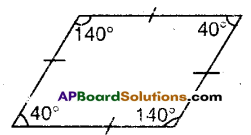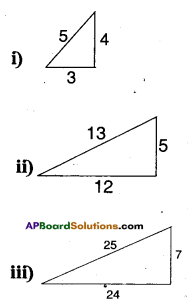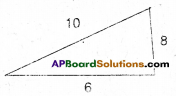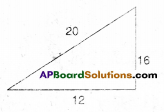# AP Board 9th Class Maths Solutions Chapter 15 Proofs in Mathematics InText Questions

AP State Syllabus AP Board 9th Class Maths Solutions Chapter 15 Proofs in Mathematics InText Questions and Answers.

## AP State Syllabus 9th Class Maths Solutions 15th Lesson Proofs in Mathematics InText QuestionsDo This

Question
Make 5 more sentences and check whether they are statements or not ? * Give reasons. [Page No. 311]
Solution:
1) 9 is a prime number – False
This is a statement because we can judge the truthness of this sentence. Clearly it is a false statement as 9 has factors other than 1 and 9, hence it is a composite number.
2) x is less than 5 – can’t say True or False
This is not a statement. The truthness can t be verified unless the value of x is known. Hence it is a sentence only.
3) 3 + 5 = 8 – True
The above sentence is a statement. It is a true statement as 5 + 3 = 8.
4) Sum of two odd numbers is even – True
The above sentence can be verified as a true sentence by taking ex¬amples like 3 + 5 = 8, 5 + 7 = 12 etc. Hence it is a true statement.
5) $$\frac{\mathrm{X}}{2}$$ +3 = 9- can’t say True or False.
The above sentence is not a state¬ment. Its truthness can’t be
verified without the value of x.Try This

1. 3 is a prime number.
2. Product of two odd integers is even.
3. For any real number x; 4x T x = 5x
4. The earth has one moon.
5. Ramu is a good driver.
6. Bhaskara has written a book “Leelavathi ”.
7. All even numbers are composite.
8. A rhombus is a square.
9. x > 7.
10. 4 and 5 are relative primes.
11. Silver fish is made of silver.
12. Humans are meant to rule the earth.
13. For any real number .v. 2x > x.
14. Havana is the capital of Cuba.

Question
Which of the above statements can be tested by giving counter example ?
[Page No. 312]
Solution:
Statements 2, 7, 8, 13 can be tested by giving counter examples 2) Product of two odd integers is even. Counter example.
2) Product of two odd integers 3 and 5 is 3×5 = 15 is not an even number.
7) All even numbers are composite. Counter example : 2 is an even prime.
8) A rhombus is a square.Counter example: (40°, 140°, 40°. 140°) is a rhombus.
13) For anyx; 2x > x
Counter example : for x = -3:
2x = 2(-3) = – 6
here -6 < – 3b

Try This

Envied by the popularity of Pythagoras, his younger brother claimed a different relation between the sides of a right angled triangles. [Page No. 319]Liethagoras Theorem: In any right angled triangle the square of the smallest side equals the sum of the other sides. Check this conjecture, whether It is right or wrong.
Solution:
This conjecture is true for the above
triangles.
i) 32 5 + 4 ⇒ 9 = 5 + 4
ii) 52 = 25 = 12 + 13
iii) 72 = 49 = 24 + 25
But, when the smallest side happens to be an even integer the conectiire may not hods good.
Eg: 1) 62 = 36 ≠ 10 + 8ii) 122 = 144 ≠ 20 + 16